Anúncio

# Instruction 7.pptx

HebaEng
23 de Mar de 2023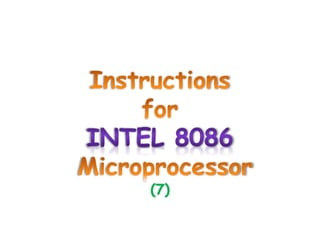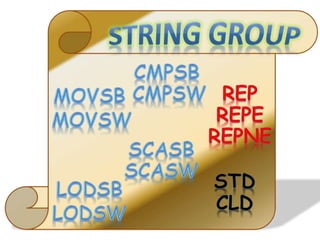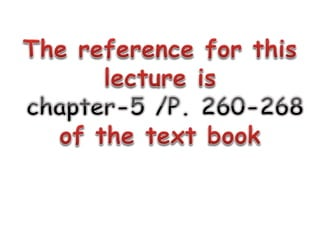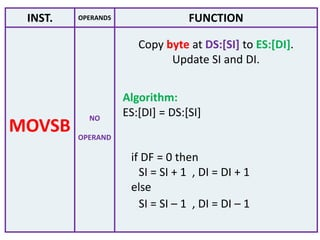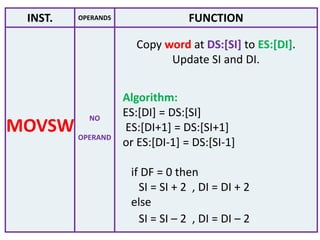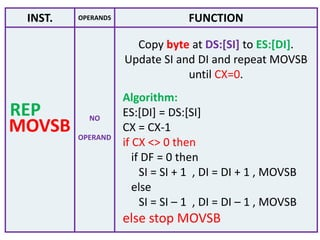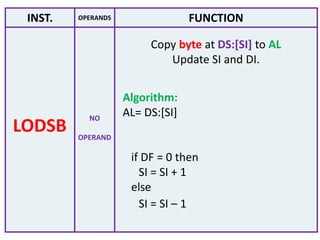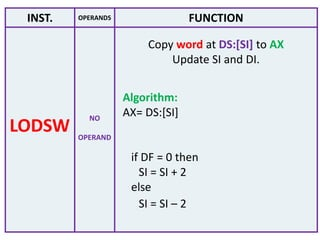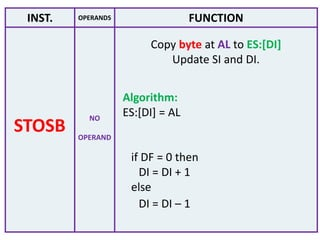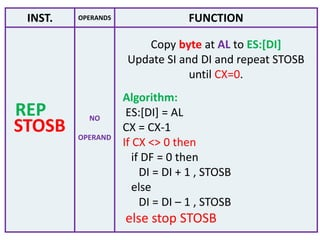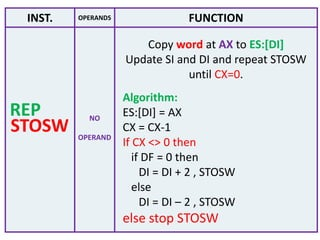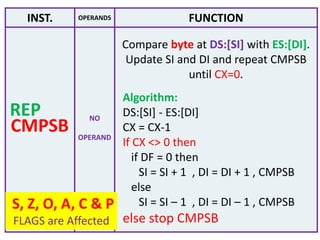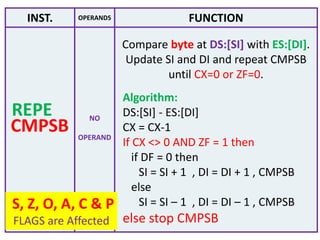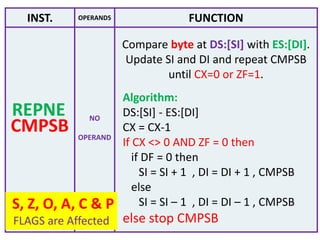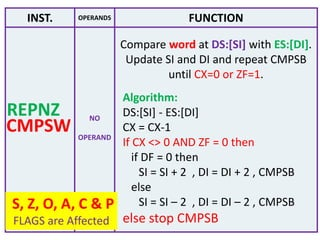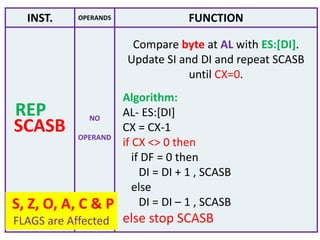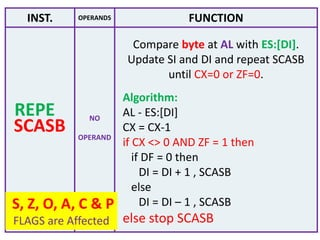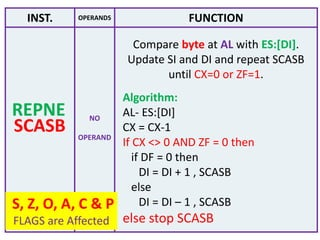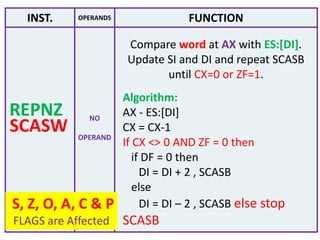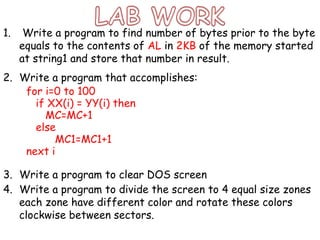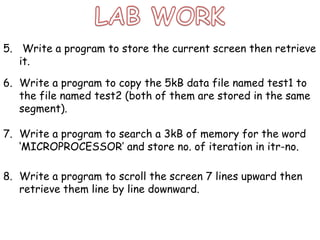1 de 24
Anúncio

### Instruction 7.pptx

1. (7)
2. INST. OPERANDS FUNCTION NO OPERAND Copy byte at DS:[SI] to ES:[DI]. Update SI and DI. MOVSB Algorithm: ES:[DI] = DS:[SI] if DF = 0 then SI = SI + 1 , DI = DI + 1 else SI = SI – 1 , DI = DI – 1
3. INST. OPERANDS FUNCTION NO OPERAND Copy word at DS:[SI] to ES:[DI]. Update SI and DI. MOVSW Algorithm: ES:[DI] = DS:[SI] ES:[DI+1] = DS:[SI+1] or ES:[DI-1] = DS:[SI-1] if DF = 0 then SI = SI + 2 , DI = DI + 2 else SI = SI – 2 , DI = DI – 2
4. INST. OPERANDS FUNCTION NO OPERAND Copy byte at DS:[SI] to ES:[DI]. Update SI and DI. MOVSB Algorithm: ES:[DI] = DS:[SI] if DF = 0 then SI = SI + 1 , DI = DI + 1 else SI = SI – 1 , DI = DI – 1
5. INST. OPERANDS FUNCTION NO OPERAND Copy byte at DS:[SI] to ES:[DI]. Update SI and DI and repeat MOVSB until CX=0. MOVSB Algorithm: ES:[DI] = DS:[SI] CX = CX-1 if CX <> 0 then if DF = 0 then SI = SI + 1 , DI = DI + 1 , MOVSB else SI = SI – 1 , DI = DI – 1 , MOVSB else stop MOVSB REP
6. INST. OPERANDS FUNCTION NO OPERAND Copy byte at DS:[SI] to AL Update SI and DI. LODSB Algorithm: AL= DS:[SI] if DF = 0 then SI = SI + 1 else SI = SI – 1
7. INST. OPERANDS FUNCTION NO OPERAND Copy word at DS:[SI] to AX Update SI and DI. LODSW Algorithm: AX= DS:[SI] if DF = 0 then SI = SI + 2 else SI = SI – 2
8. INST. OPERANDS FUNCTION NO OPERAND Copy byte at AL to ES:[DI] Update SI and DI. STOSB Algorithm: ES:[DI] = AL if DF = 0 then DI = DI + 1 else DI = DI – 1
9. INST. OPERANDS FUNCTION NO OPERAND Copy byte at AL to ES:[DI] Update SI and DI and repeat STOSB until CX=0. STOSB Algorithm: ES:[DI] = AL CX = CX-1 If CX <> 0 then if DF = 0 then DI = DI + 1 , STOSB else DI = DI – 1 , STOSB else stop STOSB REP
10. INST. OPERANDS FUNCTION NO OPERAND Copy word at AX to ES:[DI] Update SI and DI and repeat STOSW until CX=0. STOSW Algorithm: ES:[DI] = AX CX = CX-1 If CX <> 0 then if DF = 0 then DI = DI + 2 , STOSW else DI = DI – 2 , STOSW else stop STOSW REP
11. INST. OPERANDS FUNCTION NO OPERAND Compare byte at DS:[SI] with ES:[DI]. Update SI and DI and repeat CMPSB until CX=0. CMPSB Algorithm: DS:[SI] - ES:[DI] CX = CX-1 If CX <> 0 then if DF = 0 then SI = SI + 1 , DI = DI + 1 , CMPSB else SI = SI – 1 , DI = DI – 1 , CMPSB else stop CMPSB REP S, Z, O, A, C & P FLAGS are Affected
12. INST. OPERANDS FUNCTION NO OPERAND Compare byte at DS:[SI] with ES:[DI]. Update SI and DI and repeat CMPSB until CX=0 or ZF=0. CMPSB Algorithm: DS:[SI] - ES:[DI] CX = CX-1 If CX <> 0 AND ZF = 1 then if DF = 0 then SI = SI + 1 , DI = DI + 1 , CMPSB else SI = SI – 1 , DI = DI – 1 , CMPSB else stop CMPSB REPE S, Z, O, A, C & P FLAGS are Affected
13. INST. OPERANDS FUNCTION NO OPERAND Compare byte at DS:[SI] with ES:[DI]. Update SI and DI and repeat CMPSB until CX=0 or ZF=1. CMPSB Algorithm: DS:[SI] - ES:[DI] CX = CX-1 If CX <> 0 AND ZF = 0 then if DF = 0 then SI = SI + 1 , DI = DI + 1 , CMPSB else SI = SI – 1 , DI = DI – 1 , CMPSB else stop CMPSB REPNE S, Z, O, A, C & P FLAGS are Affected
14. INST. OPERANDS FUNCTION NO OPERAND Compare word at DS:[SI] with ES:[DI]. Update SI and DI and repeat CMPSB until CX=0 or ZF=1. CMPSW Algorithm: DS:[SI] - ES:[DI] CX = CX-1 If CX <> 0 AND ZF = 0 then if DF = 0 then SI = SI + 2 , DI = DI + 2 , CMPSB else SI = SI – 2 , DI = DI – 2 , CMPSB else stop CMPSB REPNZ S, Z, O, A, C & P FLAGS are Affected
15. INST. OPERANDS FUNCTION NO OPERAND Compare byte at AL with ES:[DI]. Update SI and DI and repeat SCASB until CX=0. SCASB Algorithm: AL- ES:[DI] CX = CX-1 if CX <> 0 then if DF = 0 then DI = DI + 1 , SCASB else DI = DI – 1 , SCASB else stop SCASB REP S, Z, O, A, C & P FLAGS are Affected
16. INST. OPERANDS FUNCTION NO OPERAND Compare byte at AL with ES:[DI]. Update SI and DI and repeat SCASB until CX=0 or ZF=0. SCASB Algorithm: AL - ES:[DI] CX = CX-1 if CX <> 0 AND ZF = 1 then if DF = 0 then DI = DI + 1 , SCASB else DI = DI – 1 , SCASB else stop SCASB REPE S, Z, O, A, C & P FLAGS are Affected
17. INST. OPERANDS FUNCTION NO OPERAND Compare byte at AL with ES:[DI]. Update SI and DI and repeat SCASB until CX=0 or ZF=1. SCASB Algorithm: AL- ES:[DI] CX = CX-1 If CX <> 0 AND ZF = 0 then if DF = 0 then DI = DI + 1 , SCASB else DI = DI – 1 , SCASB else stop SCASB REPNE S, Z, O, A, C & P FLAGS are Affected
18. INST. OPERANDS FUNCTION NO OPERAND Compare word at AX with ES:[DI]. Update SI and DI and repeat SCASB until CX=0 or ZF=1. SCASW Algorithm: AX - ES:[DI] CX = CX-1 If CX <> 0 AND ZF = 0 then if DF = 0 then DI = DI + 2 , SCASB else DI = DI – 2 , SCASB else stop SCASB REPNZ S, Z, O, A, C & P FLAGS are Affected
19. MOV AX, code MOV DS,AX MOV ES,AX MOV DI,OFFSET dest LEA SI,srs MOV CX,ssize CLD REP MOVSB HLT Dest db 1000 dup (?) SrS db 1000 dup (?) Ssize dw 50 MOV AX, code MOV DS,AX MOV AX,EXTRA MOV ES,AX LEA DI,ES:[dest] MOV SI,OFFSET DS:[srs] MOV CX,DS:[ssize] CLD REP MOVSW HLT Srs db 1000 dup (?) Ssize dw 50 EXTRA SEGMENT Dest db 1000 dup (?) EXTRA ENDS
20. 1. Write a program to find number of bytes prior to the byte equals to the contents of AL in 2KB of the memory started at string1 and store that number in result. 2. Write a program that accomplishes: for i=0 to 100 if XX(i) = YY(i) then MC=MC+1 else MC1=MC1+1 next i 3. Write a program to clear DOS screen 4. Write a program to divide the screen to 4 equal size zones each zone have different color and rotate these colors clockwise between sectors.
21. 5. Write a program to store the current screen then retrieve it. 6. Write a program to copy the 5kB data file named test1 to the file named test2 (both of them are stored in the same segment). 7. Write a program to search a 3kB of memory for the word ‘MICROPROCESSOR’ and store no. of iteration in itr-no. 8. Write a program to scroll the screen 7 lines upward then retrieve them line by line downward.
Anúncio